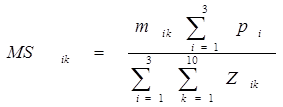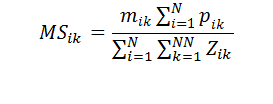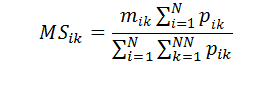# Formula (math) notationon
Experts:

I need someone to validate (and potentially correct) "math notation" for a formula.

Please see attached XLS which illustrates the process.

EEH
Math-Notation.xls
Comment
Watch Question

Do more withEXPERT OFFICE® is a registered trademark of EXPERTS EXCHANGE®
Mechanical Engineer
Most Valuable Expert 2013
Top Expert 2013

Commented:
I'm using __ to represent subscripting in the following discussion.

I think it better to refer to Z_ik as the response by Participant_i about Process_k. You then need to sum Z_ik over both the Participants and Processes. So you need a double summation.

Likewise, you should be referring to MS_ik and m_ik for the Modified Score and Participating rating factor for Participant_i and Process_k

You should also get rid of the asterisk--that's used by Excel formulas to imply multiplication, but isn't really used in mathematical representations.

I wish that the Formula Editor displayed the subscripts closer to the variables.Analyst

Commented:
byundt:

Awesome... that looks much more representative of the process.

I forgot to mention only one thing...  there will be n number of participants (this is for a survey).   So, the "3" shouldn't be hard-coded.

Likewise, instead of hard-coding "10", it might be best to also use a variable.   That is, organization X might have 10 processes, but organization Y may have smaller or larger (unknown) number.

So, how would the notation look change given that I don't always have "3" and "10".   Sorry, I should have mentioned that important component.

EEH
Analyst

Commented:
byundt:

Again, thanks so much for your response.   I'm almost "there".

Please see my response above... and attached Word 2003 file.   Equation Editor works a bit different there.

Based on my question above/last post, do the changes accurately reflect how the notation should be written?   Again, see attached .doc.

EEH
Formula.doc
Mechanical Engineer
Most Valuable Expert 2013
Top Expert 2013

Commented:
I redid the Equation using Excel 2013 using N for the number of Participants and NN for the number of Processes. I also realized that process participation p_ik needed to be subscripted both for Participant and Process.

Note that the equation editor was dramatically improved in Excel 2010.Analyst

Commented:
Okay... that looks great:

So, let me make sure I get the new definitions right:

N = Number of Participants
NN = Number of Processes
i = Counter for N
k = Counter for NN

Is that correct?

EEH
Analyst

Commented:
Again, thanks... I posted my question too fast.

Quick follow-up...  in my actual process, I also evaluate three additional criteria.
The above example describes it for "modified".   I also have "r", "s", and "d" that will be evaluate.   Obviously, that's just plug-n-play.

AND, there's a cumulative effect (product of m * r * S *d).   My final question, do I merely replace any instance of "m" with "mrsd" in the notation?

I hope that would do it.  Again, I truly appreciate your assistance in this!!!   8)

EEH
Mechanical Engineer
Most Valuable Expert 2013
Top Expert 2013
Commented:
After doing some more thinking, I question whether Z is needed at all. Really, you are using the same p_ik in the bottom summations as in the top one.where:
MS_ik = Modified Score
i = Participant subscript
N= Number of Participants
k = Process subscript
NN = Number of Processes
m_ik = Weighting factor assigned by Participant i for Process k
p_ik = Whether Participant i supports Process k
FormulaQ28104150.doc
Mechanical Engineer
Most Valuable Expert 2013
Top Expert 2013

Commented:
N = Number of Participants
NN = Number of Processes
i = Counter for N
k = Counter for NN

Classical math would use a single letter to represent each variable. You could then use mrsd in an Equation, and everyone would understand that multiplication is required. Had you not already used m for a variable, I'd have suggested M and N for the numbers of Processes and Participants.

Given that we are already using a mix of one and two-letter symbols for variables, you will need to add separation, subscripting, parentheses or asterisks between variables to imply multiplication.
m r s d
m_ikr s d
m_ik(r s d)
Analyst

Commented:
Ok... I hear you... and believe you.

If I were to explain this, I can easily describe the numerator part and identify the "7" and "1" (following the conceptual model in XLS).

For the denominator part, I'm not sure how I get to the "12"... don't the bottom terms infer multiplication across N and NN?   Or am I mixing something up?

EEH
Analyst

Commented:
Thanks for the last response on the modified equation.   See my reply above.

Hmh, for the other question, you wrote:
m_ik(r s d)

I would have thought mrsd_ik.   Right or wrong?

EEH
Analyst

Commented:
byundt:

I think I'm good... again, not sure if there's a difference between "m_ik(r s d)" or "mrsd_ik".

But for right now, you've definitely answered the original question.

The moment I saw that you replied to the post, I knew I was in good hands.

Thanks again!

EEH
Analyst

Commented:
More than excellent!!!!
Most Valuable Expert 2014
Top Expert 2015

Commented:
where:
MS_ik = Modified Score
i = Participant subscript
N= Number of Participants
k = Process subscript
NN = Number of Processes
m_ik = Weighting factor assigned by Participant i for Process k
p_ik = Whether Participant i supports Process k
This is notationally confusing.
Does the i in p_ik represent the i from MS_ik, or does it represent 1,2,...,N in the sum from 1 to N?
Analyst

Commented:
ozo:

Thanks for chiming in... I think I'm good w/ byundt's explanation.   However, if this is confusing to you, it may be confusing to others.

Instead of using notation reference, let me simply summarize the process:

- A survey is distributed to a large population.   An unknown number of participants (N) will respond to it.
- There will be different survey for different organizations (as part of the research).   Org X may have 10 processes (NN) while Org Y may have 8 processes (NN).
- Each survey repondent (whether in Org X or Org Y) will have to rate four (4) criteria.   Let's just abbreviate them as: M, R, S, and D
- The orginal question (to simplify it) focused on the notation for "M" only.   In essense, all four criteria are being computed in the same fashion (so the same notation will apply... I merely update the variable references).
- And finally, there will be an overall criteria.   That however is computed (not provided via survey) as a result of the survey responses for the four criteria.    This "MRSD" overall score will follow the same principle except that it takes the product (M*R*S*D).
- So, again, the process applies except that it's not take a single value but the product of four values before summing up "p_ik" and summing up all selected processes.

Makes sense?   Let me know if you'd have an additional viewpoint on this.   Obviously, I'd like to get the notation right.   (My undergrad is NOT in math... so reading notation is "semi-Greek" to me).

Thanks,
EEH
Most Valuable Expert 2014
Top Expert 2015

Commented:
If the i in p_ik is meant to always range over 1..N regardless of the value of the i in MS_ik, then it would be notationally cleaner to use different letters for the two different subscripts.

In the denominator in your fraction is meant to be a constant total of all p_, that does not depend on which MS_ you are computing, then it may help to make it more obvious by separating that out.
On the other hand, if the p_i sum in the numerator is the same as the p_i sum in the denominator, then it may be notationally clearer to reverse the order of the summations in the denominator so that the sum from 1 to N is inside the sum from 1 to NN
which would allow separating out the sum in the numerator.
Analyst

Commented:
Ozo:

I hear you but I'm not sure if I'm fully tracking.   Would you be so kind and include your suggestions/modification to the attached Word document?

Please double-click on the equation to bring up the Equation Editor.

Thanks,
EEH
Formula.doc

Do more with